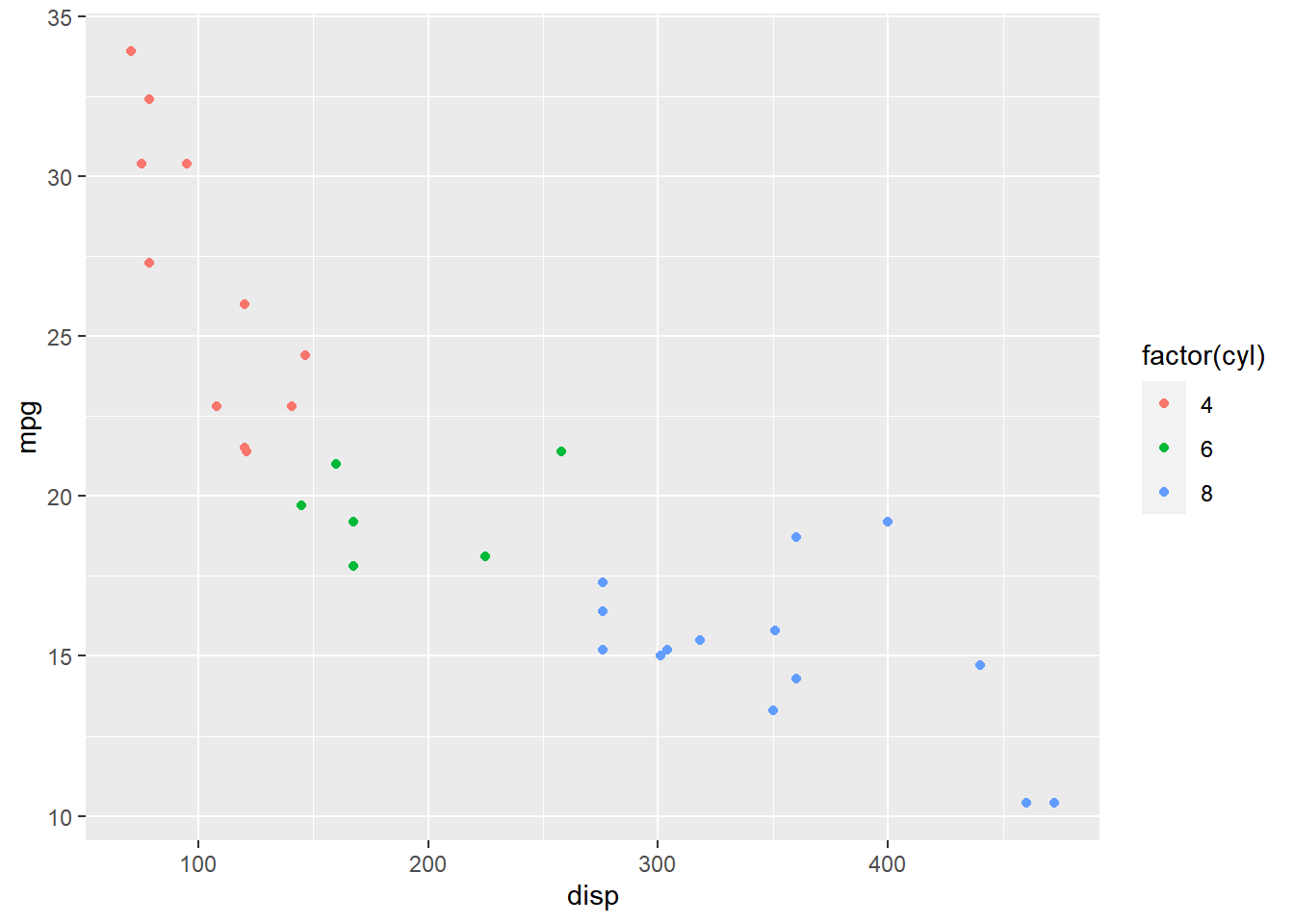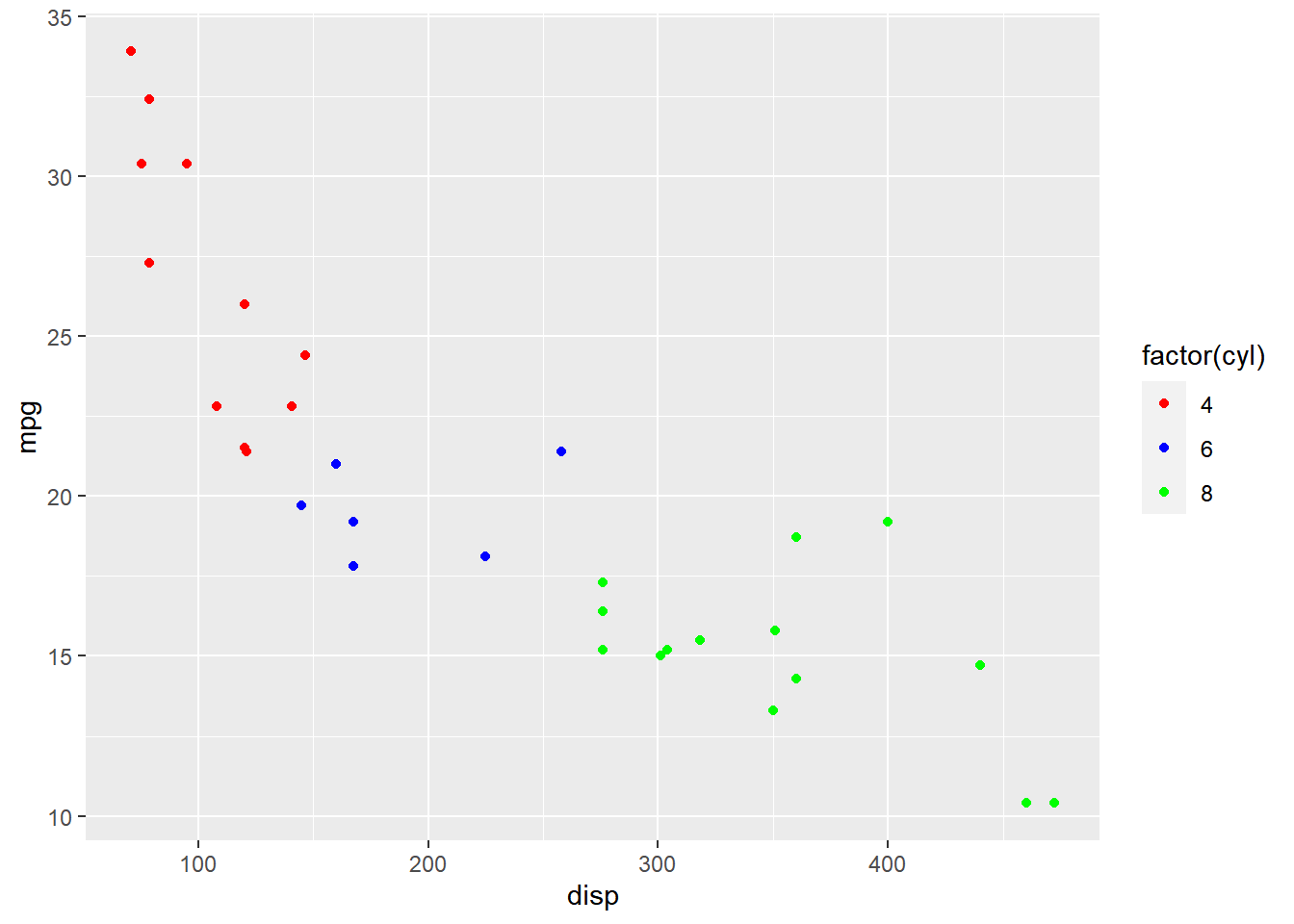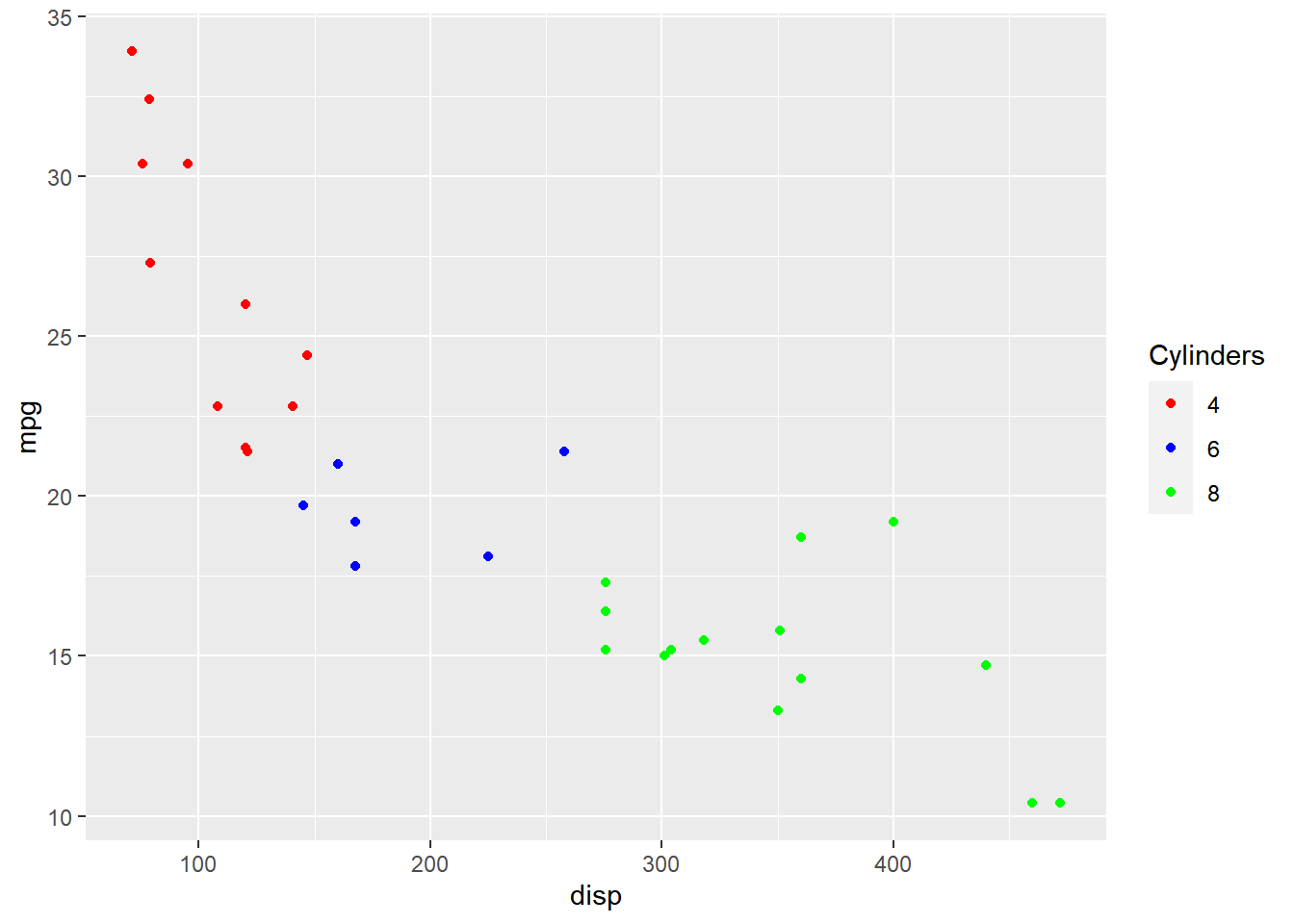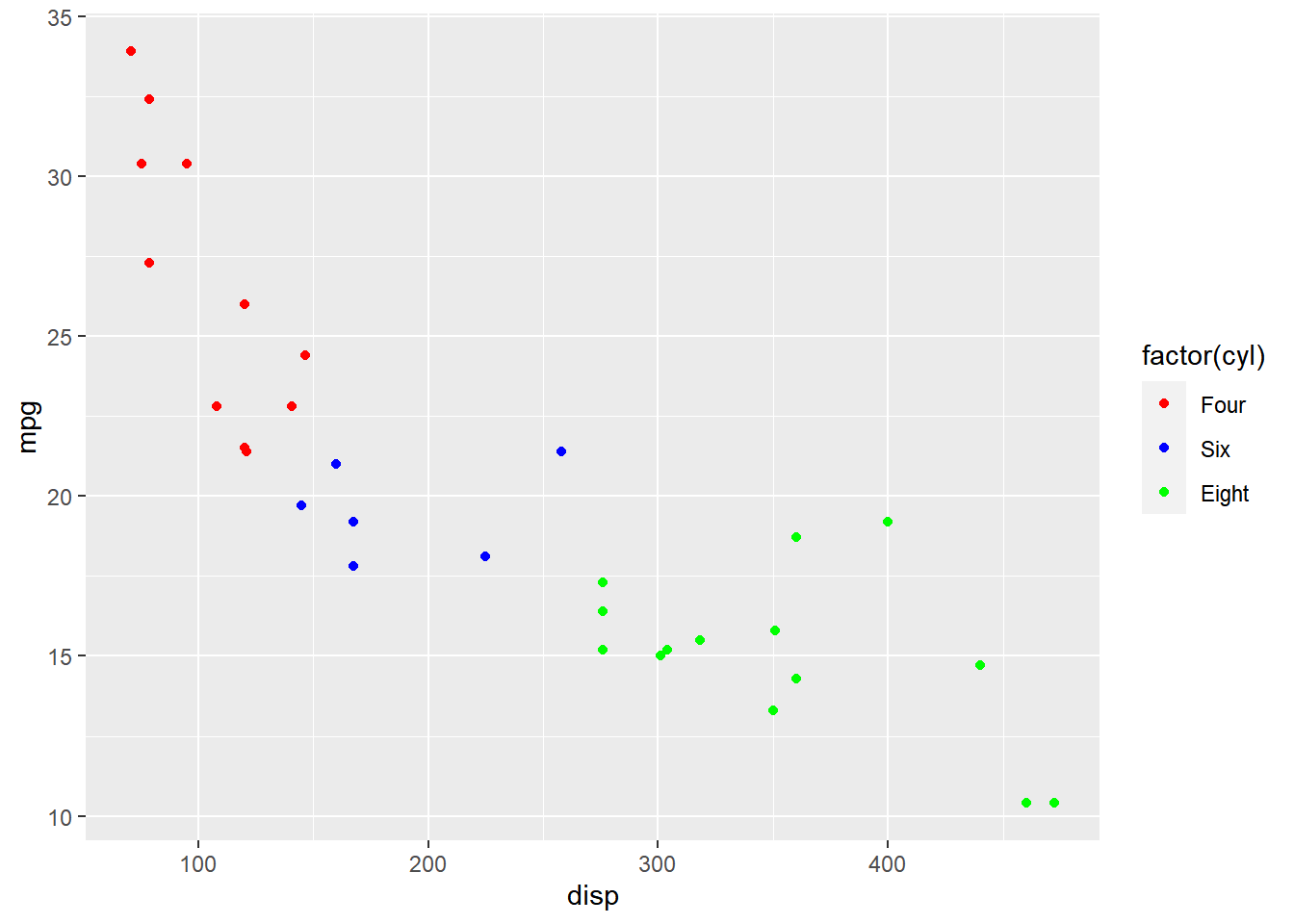Want to share your content on R-bloggers? click here if you have a blog, or here if you don't.

### Introduction

This is the 13th post in the series Elegant Data Visualization with
ggplot2
. In the previos post, we learnt how to modify the axis of plots. In
this post, we will focus on modifying the appearance of legend of plots when
the aesthetics are mapped to variables. Specifically, we will learn to modify
the following when `color` is mapped to categorical variables:

• title
• breaks
• limits
• labels
• values

### Libraries, Code & Data

We will use the following libraries in this post:

All the data sets used in this post can be found here

### Basic Plot

Let us start with a scatter plot examining the relationship between displacement
and miles per gallon from the mtcars data set. We will map the color of the points
to the `cyl` variable.

``````ggplot(mtcars) +
geom_point(aes(disp, mpg, color = factor(cyl)))``````As you can see, the legend acts as a guide for the `color` aesthetic. Now, let
us learn to modify the different aspects of the legend.

### Values

To change the default colors in the legend, use the `values` argument and
supply a character vector of color names. The number of colors specified
must be equal to the number of levels in the categorical variable mapped.
In the below example, `cyl` has 3 levels (4, 6, 8) and hence we have specified
3 colors.

``````ggplot(mtcars) +
geom_point(aes(disp, mpg, color = factor(cyl))) +
scale_color_manual(values = c("red", "blue", "green"))``````### Title

In the previous example, the title of the legend (`factor(cyl)`) is not very
intuitive. If the user does not know the underlying data, they will not be able
to make any sense out of it. Let us change it to `Cylinders` using the `name`
argument.

``````ggplot(mtcars) +
geom_point(aes(disp, mpg, color = factor(cyl))) +
scale_color_manual(name = "Cylinders",
values = c("red", "blue", "green"))``````Now, the user will know that the different colors represent number of cylinders in
the car.

### Limits

Let us assume that we want to modify the data to be displayed i.e. instead of
examining the relationship between mileage and displacement for all cars, we
desire to look at only cars with at least 6 cylinders. One way to approach this
would be to filter the data using `filter` from dplyr and then visualize it.
Instead, we will use the `limits` argument and filter the data for visualization.

``````ggplot(mtcars) +
geom_point(aes(disp, mpg, color = factor(cyl))) +
scale_color_manual(values = c("red", "blue", "green"), limits = c(6, 8))``````
``## Warning: Removed 11 rows containing missing values (geom_point).``As you can see above, `ggplot2` returns a warning message indicating data related
to 4 cylinders has been dropped. If you observe the legend, it now represents
only 4 and 6 cylinders.

### Labels

The labels in the legend can be modified using the `labels` argument. Let us
change the labels to `Four`, `Six` and `Eight` in the next example. Ensure that
the labels are intuitive and easy to interpret for the end user of the plot.

``````ggplot(mtcars) +
geom_point(aes(disp, mpg, color = factor(cyl))) +
scale_color_manual(values = c("red", "blue", "green"),
labels = c('Four', 'Six', 'Eight'))``````### Breaks

When there are large number of levels in the mapped variable, you may not
want the labels in the legend to represent all of them. In such cases, we can
use the breaks argument and specify the labels to be used. In the below case,
we use the `breaks` argument to ensure that the labels in legend represent
two levels (4, 8) of the mapped variable.

``````ggplot(mtcars) +
geom_point(aes(disp, mpg, color = factor(cyl))) +
scale_color_manual(values = c("red", "blue", "green"),
breaks = c(4, 8))``````### Putting it all together…

``````ggplot(mtcars) +
geom_point(aes(disp, mpg, color = factor(cyl))) +
scale_color_manual(name = "Cylinders", values = c("red", "blue", "green"),
labels = c('Four', 'Six', 'Eight'), limits = c(4, 6, 8), breaks = c(4, 6, 8))``````### Summary

In this post, we learnt to modify the following aspects of legends:

• title
• breaks
• limits
• labels
• values

### Up Next..

In the next post, we will learn how to modify legend when `fill` is mapped to variables.